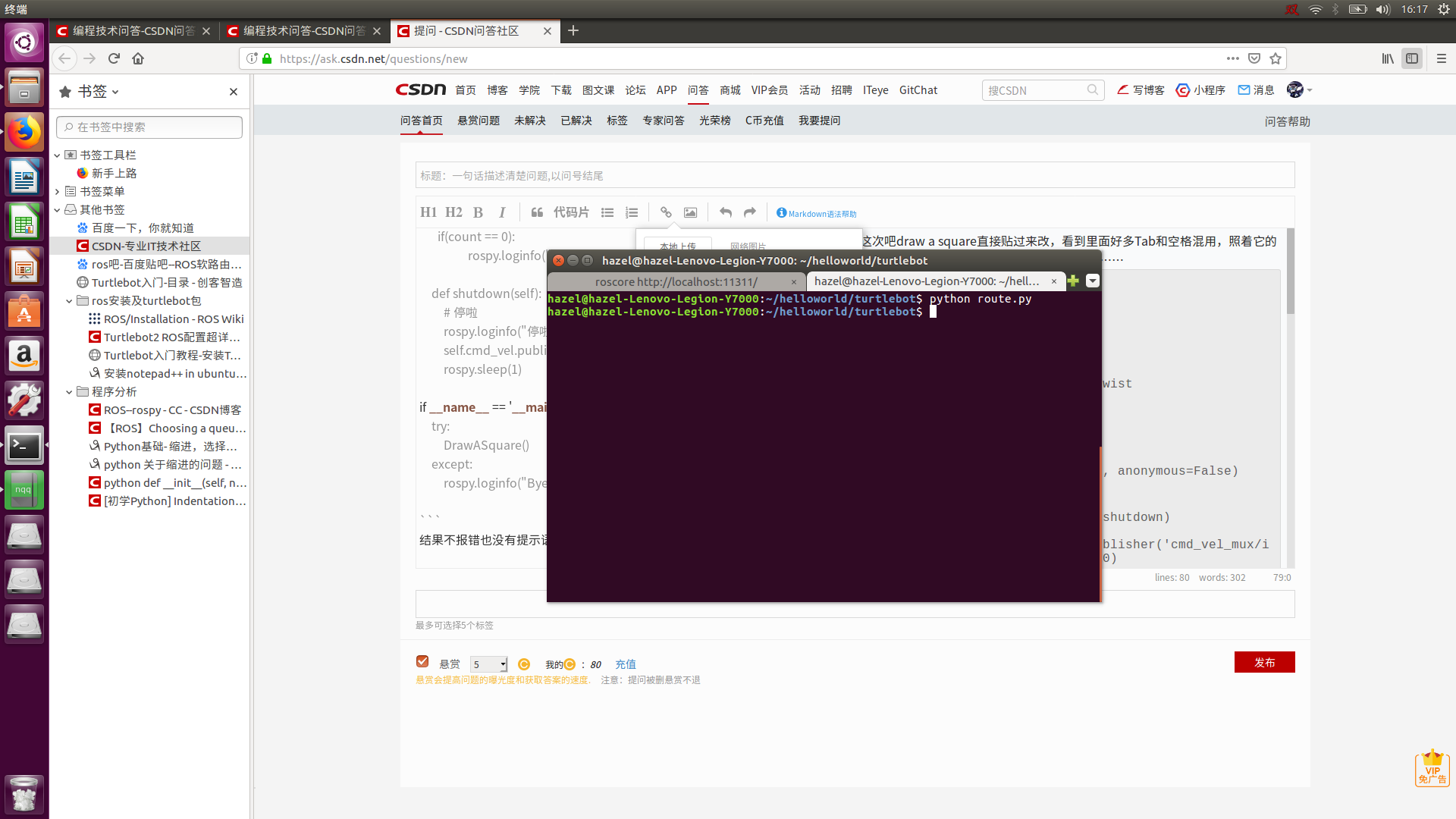``````# -*- coding: UTF-8 -*-
#!/usr/bin/env python

#前进1m，画边长（ 0.5 ）m的五边形

import rospy
from geometry_msgs.msg import Twist

class ROUTE():
def __init__(self):
# 初始化节点
rospy.init_node('route', anonymous=False)

# ctrl + c退出
rospy.on_shutdown(self.shutdown)

self.cmd_vel = rospy.Publisher('cmd_vel_mux/input/navi', Twist, queue_size=10)

# 5 HZ更新频率
r = rospy.Rate(5);

# 两个不同的Twist，转，直走

# 0.5 m/s 前进
move_cmd = Twist()
move_cmd.linear.x = 0.5

#旋转18 deg/s
turn_cmd = Twist()
turn_cmd.linear.x = 0

count = 0
# 前进1M
for x in range(0,10):
self.cmd_vel.publish(move_cmd)
r.sleep()
while not rospy.is_shutdown():
#转54度
for x in range(0,15):
self.cmd_vel.publish(turn_cmd)
r.sleep()
# 前进0.5M
for x in range(0,5):
self.cmd_vel.publish(move_cmd)
r.sleep()
# 转234度
for x in range(0,65):
self.cmd_vel.publish(turn_cmd)
r.sleep()
count = count + 1
if(count == 5):
count = 0
if(count == 0):

def shutdown(self):
# 停啦
self.cmd_vel.publish(Twist())
rospy.sleep(1)

if __name__ == '__main__':
try:
DrawASquare()
except:

``````2个回答

# -*- coding: UTF-8 -*-

#!/usr/bin/env python

#前进1m，画边长（ 0.5 ）m的五边形

import rospy
from geometry_msgs.msg import Twist

class ROUTE():
def init(self):
# 初始化节点
rospy.init_node('route', anonymous=False)

``````    # ctrl + c退出
rospy.on_shutdown(self.shutdown)

self.cmd_vel = rospy.Publisher('cmd_vel_mux/input/navi', Twist, queue_size=10)

# 5 HZ更新频率
r = rospy.Rate(5);

# 两个不同的Twist，转，直走

# 0.5 m/s 前进
move_cmd = Twist()
move_cmd.linear.x = 0.5

#旋转18 deg/s
turn_cmd = Twist()
turn_cmd.linear.x = 0

count = 0
# 前进1M
for x in range(0,10):
self.cmd_vel.publish(move_cmd)
r.sleep()
while not rospy.is_shutdown():
#转54度
for x in range(0,15):
self.cmd_vel.publish(turn_cmd)
r.sleep()
# 前进0.5M
for x in range(0,5):
self.cmd_vel.publish(move_cmd)
r.sleep()
# 转234度
for x in range(0,65):
self.cmd_vel.publish(turn_cmd)
r.sleep()
count = count + 1
if(count == 5):
count = 0
if(count == 0):

def shutdown(self):
# 停啦
self.cmd_vel.publish(Twist())
rospy.sleep(1)
``````

if name == '__main__':
try:
DrawASquare()
except:

``````大致帮你缩进了一下，试试行不行。

for 语句循环语句需要相对于for行有缩进，while也同样。
``````
``````    1、缩进有问题，一个函数内的缩进应该是一致的。以下预计应增加缩进。
count = 0
# 前进1M